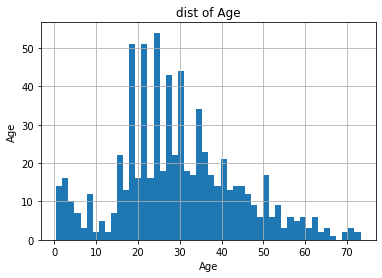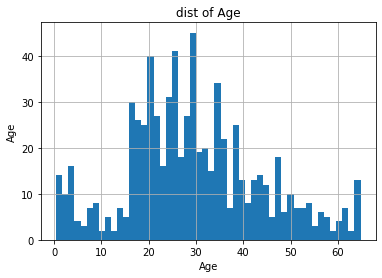# Techniques to detect and handle outliers in dataset | Hands-on feature engineering Part-V

Hello and welcome to the wonderful series of Feature engineering where we are exploring different techniques to prepare, and preprocess our data in the best possible way to extract the most out of it. In some of our previous blogs of this series we have studied the techniques to impute missing values, feature transformation techniques, feature scaling techniques, categorical encodings, and in this tutorial, we will discuss how to detect and handle outliers.

## What are Outliers?

Outliers are the data points that are significantly different from the remaining data. It means that outliers deviate from the relationship of variables and it affects greatly the statistical measurements like mean, median.

suppose for example if we have 5 data points as 2,4,5,3,7 and if we find mean it is 4.8, But suppose that if it consists of one more point which is 99 and then the mean of it it is 20. what major change in mean you have observed? It is only due to a single point that is far from the initial scale of data, and this single point(99) is only known as an Outlier.

## How outliers are introduced in datasets?

• Human error - Data is collected through a different survey where most people fill wrong values so outliers may be due to human error.
• Measurement error - Data is also collected through readings by measuring instrument and due to certain fault instrument can give faulty results.
• Data processing errors - The data you received can be secondary like before to you someone has worked on it and during preprocessing made some errors.

• Sampling error - Mixing the data with incorrect values or the wrong sample rates can also produce outliers.
• Intentional Error - Sometimes dummy outliers are created to test some test cases.

## Why dealing with outliers is important in machine learning?

Outliers are present in very few amounts in the dataset but can greatly affect the machine learning model performance. Let us discuss each factor point-wise to understand clearly why we should remove the outliers or change them to a common scale.

• The actual source of the presence of outlier in data is not clear. And while performing different data preprocessing or statistical calculations outliers will produce unwanted results as we have seen above.
• Outliers have a major impact on linear algorithms like linear regression, Logistic Regression, Adaboost, and on all deep learning techniques. Common this among all these techniques is they work on weights so think when you are working on modeling that the algorithm is weight-based or not.
• And on the Other side outliers do not impact tree-based algorithms like Decision Tree, Random Forest, etc.

## How to Detect Outliers?

Now the question arises that if they are very few amounts of outliers in a large dataset then how to find where is the presence of outliers in the dataset? So, data visualization techniques proved to provide better and more accurate results where you can visualize the outliers in various plots. major used technique to detect outliers is listed below.

• Using Visualizing plots such as boxplot, scatter plot.
• using the Normal Distribution(mean and standard deviation).

#### Dataset Overview

We will be using the same Titanic Dataset for studying and demonstrating each technique to detect and handle outliers. If you are following the feature engineering series then you must be known about the titanic data set and have downloaded it. If not you can easily find the dataset from this link or on Kaggle. If you do not want to download the dataset then simply create a Kaggle notebook of the same dataset and practice each technique.

👉 let us pick the "AGE" variable from the dataset and find if there an outliers present.

#### 1) Using Histogram

The histogram is a simple plot that helps to visualize the distribution of a variable.

#### 2) Using BoxPlot

Observations
• From both the plots as boxplot and distribution plot we can clearly see that there is the presence of some outliers at the end.
• we can observe that the mean age of people is 29.
• It is showing that the 25th(1st) quantile lies at age 20, 50th(2nd) quantile at 29, 75th(3rd) at 38 and also shows that age above 68 is outliers in a dataset.

Now, let's explore various techniques to Handle such outliers. we should also take care of variable distribution while dealing with outliers.

## Methods to Handle Outliers

There are various techniques for handling outliers that are used according to the problem statement that we aim to solve. so let's get started with each technique and understand which one best suits our problem statement.

#### 1) Trimming

Trimming simply refers to removing all the outliers and getting rid of them. But this technique is not useful in most of the use cases and when you have less data or you are working on a problem where each point has its own importance in production then it is not suggested to use this technique.

To apply this technique we only need to decide if the metric above or below which we need to remove all data points. It means we need to find the lower boundary and upper boundary in which we want to keep the data else get rid of it. We can use IQR(Interquartile range) to find the lower and upper boundaries.

IQR = 3rd Quantile - 1st Quantile

lower_bound   =   1st quantile  -  1.5  * IQR
upper_bound  =   3rd quantile + 1.5  * IQR

Key Points
• a simple and straightforward technique
• easy to implement
• whenever you have a very large dataset and outliers are in very little quantity then you can easily use this technique.

#### 2) CENSORING

Censoring also known as capping is a technique of setting a maximum and/or a minimum of distribution at an arbitrary value. It simply means the values which are out of range get replaced by these values.

Key Points
• It does not remove any kind of data(no loss of data).
• It distorts the shape of the distribution of variables.

Capping can be done using various methods which we are going to discuss as follows.

I) Gaussian Approximation

If the data is normally distributed then we can use this technique to handle outliers. To calculate the upper and lower boundary we will use the same IQR but instead of multiplying by 1.5, we will multiply by 3 to get extreme ends.

Code:

Now after applying this technique we can see the distribution of a variable. The code is the same as we use above in the histogram method.The difference between the change in outliers can be easily observed between this graph and the above-plotted graph.

II) Inter-quantal range proximity rule

In this technique, boundaries are determined using the Inter-quantile range(IQR) which is the same as we saw in trimming. Only the difference is there we remove the values above and below that boundaries whereas here we map the values with upper and lower boundaries calculated which are greater or less than these boundary-values.

Code:

Let's see what change is observed in the shape of a distribution. the code is the same for plotting the distribution plot.#### III) Arbitrarily

The technique is very simple where you are independent to choose any upper or lower boundary value of your choice according to your observation of outliers. You can also keep outliers at different positions like for lower outliers keep them at 0 or -1 and upper at 99 or 100. This technique is used by most people to provide utmost importance to outliers. It completely depends on your choice of value according to the shape of a distribution.

code:

#### IV) Using Quantiles

The method is very similar to the above-discussed method, only the difference is that there you directly choose the upper and lower boundary of your choice whereas in this technique you can independently choose the quantiles rather than using fixed 0.25 and 0.75.

Code:

#### 3) Imputation Techniques

This is a very simple technique where we assume all the outliers as missing values and use the missing value imputation techniques to fill the outliers with the correct value of use.

👉 We have already discussed various Missing value imputation techniques at the start of this Hands-On feature engineering series. You can visit that article and study each imputation technique.

## SUMMARY

In this article, we have studied that outliers are the data points that lie at a very far range from an actual range in the dataset and present in very little quantity but hurt model performance and statistical calculations. Therefore detecting the presence of outliers in a dataset and handling it is important before jumping to the modeling task. And we have discussed different methods with a practical implementation that you can use to deal with outliers.

👉I hope that each and every technique that we have studied in this blog is easy to understand and crystal clear to you. If you have any queries then please post them in the comment section below or you can also use our contact form to send any personalized queries.

Thank You! 😊
Keep Learning, Happy Learning

1.